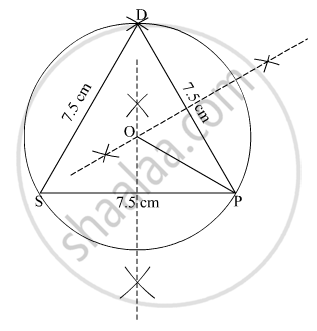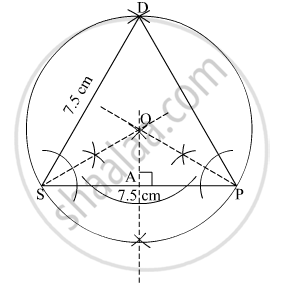Advertisement Remove all ads

# Construct Incircle and Circumcircle of an Equilateral δ Dsp with Side 7.5 Cm. Measure the Radii of Both the Circles and Find the Ratio of Radius of Circumcircle to the Radius of Incircle. - Geometry

Diagram

Construct incircle and circumcircle of an equilateral
Δ DSP with side 7.5 cm. Measure the radii of both the circles and find the ratio of radius of circumcircle to the radius of incircle.

Advertisement Remove all ads

#### Solution

Steps of construction:
1. Draw a line SP = 7.5 cm.
2. With S as centre and 7.5 cm as radius, draw an arc above the line SP.
3. With P as centre and 7.5 cm as radius, cut an arc on on the previous drawn arc and name the point of intersection as D.
4. Join DS and DP. Δ DSP is thus obtained.
5. Draw the perpendicular bisectors of the lines DP and SP. Let these perpendicular bisectors meet at point O.
4. With O as centre and OP as radius, construct a circle touching all the vertices of the Δ DSP.
This circle is thus the required circumcircle.
Radius = 4 cmSteps of construction
1. Draw a line SP = 7.5 cm.
2. With S as centre and 7.5 cm as radius, draw an arc above the line SP.
3. With P as centre and 7.5 cm as radius, cut an arc on on the previous drawn arc and name the point of intersection as D.
4. Join DS and DP. Δ DSP is thus obtained.
5. Draw the angle bisectors of angle S and P and let them meet at point O.
6. Draw the perpendicular from point O to the line SP. Join OA.
7. With O as centre and OA as radius, draw a circle touching all the sides of the triangle.
This is the required incircle.
Radius = 2 cm
ratio of radius of circumcircle to the radius of incircle = 4 : 2 = 2 : 1Is there an error in this question or solution?
Advertisement Remove all ads

#### APPEARS IN

Advertisement Remove all ads
Advertisement Remove all ads
Share
Notifications

View all notifications

Forgot password?